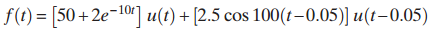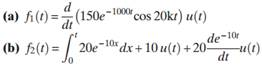Create an Account

Home / Questions / Use MATLAB to find the Laplace transform of the following waveform 2. Find the Laplace...

Use MATLAB to find the Laplace transform of the following waveform 2. Find the Laplace...

1. Use MATLAB to find the Laplace transform of the following waveform2. Find the Laplace transforms of the following waveforms.Jul 27 2020 View more View LessSubscribe To Get Solution